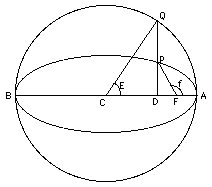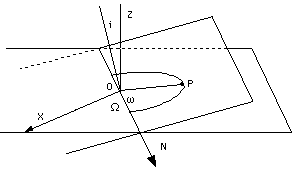# (12b) How Orbital Motion is Calculated

(Optional Section)Index10. Kepler's Laws

Kepler's Laws
(For teachers)10a. Scale of Solar Sys.11. Graphs & Ellipses11a. Ellipses
and First Law12. Second Law12a. More on 2nd Law12b. Orbital Motion12c. Venus transit (1)12d. Venus transit (2)12e. Venus transit (3)

Newtonian Mechanics13. Free Fall14. Vectors15. Energy16. Newton's Laws17. Mass
 This section looks at some finer points of planetary motion, and is primarily meant for advanced users, who may wonder how orbital motion is actually derived. For others it provides a peek at the complexity of orbital calculations, and is not required elsewhere in "Stargazers."

As stated earlier, the motion of a satellite (or of a planet)
in its elliptical orbit is given by 3 "orbital elements":

(1) The semi-major axis a, half the greatest width of the orbital ellipse, which gives the size of the orbit.

(2) The eccentricity e, a number from 0 to 1, giving the shape of the orbit. For a circle e = 0, larger values give progressively more flattened circles, up to e = 1 where the ellipse stretches to infinity and becomes a parabola. The orbits of all large planets are rather close to circles: that of Earth, for instance, has e=0.0167

(3) The mean anomaly M, an angle growing at a steady rate, increasing by 360 degrees each orbit

M = M(0) + 360°(t/T)

where M(0) is the value of M at time t=0 and T is the orbital period. Given those numbers, M is readily calculated for any time t.

However, the actual position of the satellite is given by the true anomaly φ. In polar coordinates (r,f) describing the satellite's motion in its orbital plane, f is the polar angle. The equation of the orbit is

r = a(1 – e2)/(1 + e cos φ)

The angle φ also grows by 360o each full orbit, but not at all uniformly. By Kepler's law of areas, it grows rapidly near perigee (point closest to Earth) but slowly near apogee (most distant point).

The information needed to derive φ for any time t is contained in the law of areas, but the actual calculation is not easy. The process involves an auxiliary angle, the eccentric anomaly E which like φ and M grows by 360o each orbit. At perigee, all three anomalies equal zero.The drawing on the right gives a geometric construction of those angles (no, don't try to puzzle out the details). The orbital ellipse is enclosed in a circle of radius a, and given a position P of the satellite, a corresponding point Q on the circle can be drawn, sharing the same line perpendicular to the ellipse's axis. Then E is the angle between the long axis of the ellipse and the line drawn from the center of the circle to Q ("eccentric" might mean here "from the center").

## Kepler's Equation

Suppose the elements a, e and M(0) at time t=0 are given, and we need to find the value of φ at some different time t. With f known, the above equation gives r, and (r ,φ) together pin-point the satellite's position in its orbital plane. The first step is to derive

M = M(0) + 360°(t/T)

We assume the period T is known (this requires the 3rd law and is discussed for circular orbits in sections 20 and 20a). It can then be shown that the angle E satisfies "Kepler's equation"

M = E – (180°/π)e sinE

where π = 3.14159256... is the ratio between the circumference of a circle and its diameter. How did that number suddenly crop up, you may ask? The fact is, the division of the circle into 360 degrees may be convenient to use (we inherited it from the ancient Babylonians) but the number 360 has no particular place in mathematics. It is probably related to the number of days in a year. The "natural" division of angles which arises in calculus and other branches of math is into radians, with 360 degrees equal to 2π = 6.2831... radians (making each radian equal to about 57.3 degrees). With angles measured in radians, Kepler's equation simplifies to

M = E – e sinE

No matter which form is used, mathematics knows no formula which gives E in terms of M. However, solutions can often be approximated to any degree of accuracy by iteration--by starting with an approximate solution, then improving it again and again by an appropriate procedure ("algorithm"--more about that word, here). If the eccentricity e is not too big--the ellipse not much different from a circle--then M and E are not too different. So an initial guess

E' = M

may not be too far off. Putting this guess into the term sinE gives an improved guess E"

E" = M + (180°/π)e sinE'

One can now insert E" in the sinE term and get an even closer guess, and so on and so forth... until the first (say) ten decimal digits of the value of E no longer change, at which point we may decide we have E to sufficient accuracy and stop the process. Computer handle such a process of continuous improvement ("iteration of the solution"--one form of an algorithm) very rapidly, and other methods also exist, with sufficient speed even when e is not very small.

Given E, a number of formulas will give the true anomaly φ. For instance, one can first derive

r = a(1 – e cos E)

And then cos φ can be found from

r = a(1 – e2)/(1 + e cos φ)

and sin φ follows from cos φ. All this is easily and automatically computed nowadays, but must have been a real hassle in the days before computers.

## The Orbit in SpaceThe 3 remaining orbital elements are all angles giving the position of the orbit in 3 dimensions. They are described below, but their actual use belongs to a university course in orbital mechanics and will be omitted. The angles:

1. Inclination i.
2. The argument of perigee ω (small Greek omega).
3. The longitude of the ascending node Ω (capital omega).

To orient the orbit in 3 dimensions requires a reference plane and a reference direction. For satellite orbits, the reference plane--the horizontal plane in the drawing--is usually the Earth's equatorial plane (sometimes it is the plane of the ecliptic). The reference direction in either case is the direction from the center of the Earth to the vernal equinox (which belongs to both above planes). We will call it the x direction, since that is its role in (x,y,z) coordinates used in orbital calculations.

Two non-parallel planes always intersect along a line--the way the plane of a door intersects the plane of the wall along the door's hinge. The orbital plane and the equatorial plane (used for reference) do so too, and their intersection is called the line of nodes N. Let the origin O of our coordinates be the center of the Earth, which is also the focus of the ellipse; this point belongs to both the equatorial plane and the orbital plane, and is therefore also on their intersection line N (drawing). Then...

1. The inclination i is the opening angle of the "hinge" along N. It is best defined by erecting at O lines perpendicular to each plane and measuring the angle between them (drawing).

2. The angle Ω is measured in the equatorial plane between N and the reference direction x. One can imagine rotating the "hinge" N around point O, without changing the inclination: the orbital plane then covers all possible values of Ω.
But what is this "ascending node" bit? The above definition contains some ambiguity: N defines two lines coming out of O, in opposite directions. From which of them should Ω be measured? To resolve this we note that the plane of the equator divides space into two parts, one north of it and one south of it. Specifying "the ascending node" selects the branch on which the satellite crosses as it enters the northern half-space, rather than the one crossed when leaving it.

3. Finally, ω is the angle measured in the orbital plane between N and the direction from O to the perigee point P. If perigee lies on the "hinge", on the side of positive x, then ω = 0; rotating the orbit by 90o until the line OP is perpendicular to N gives ω = 90o, rotating it further until it reaches the negative side of the x-direction gives ω = 180o.

Suppose you have the orbital elements of some satellite, e.g. the space shuttle (you can often get them off the world-wide web). The first three (a, e, M), with M given at some particular time, enable you to calculate where the satellite will be at any time in its orbit. With (i, ω, Ω) you can then find where it would be in the sky.

[For those who would like to know more about the way such rotations in space are calculated, see problem 8 in the "trigonometry proficiency drill." That problem only deals with rotations in two dimensions (e.g. on a sheet of paper), but an additional short section linked to its end explains how this is extended to 3D.]

Questions from Users:   Launch window of the Space Shuttle
***     Motion of the Moon

Optional: The 2004 Transit of Venus
12c.     Halley's Method of Deriving the AU
12d.     The displacement D of the track of Venus
12e.     Deriving the Astronomical Unit

Next Regular Stop: #13 The Way things Fall

Author and Curator:   Dr. David P. Stern
Mail to Dr.Stern:   stargaze("at" symbol)phy6.org .

Last updated: 13 October 2016